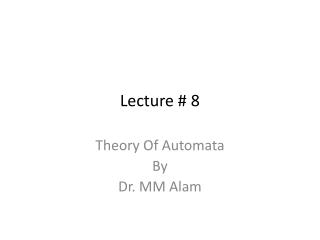# Lecture # 8 - PowerPoint PPT PresentationDownload PresentationLecture # 8

Lecture # 8Download Presentation## Lecture # 8

- - - - - - - - - - - - - - - - - - - - - - - - - - - E N D - - - - - - - - - - - - - - - - - - - - - - - - - - -
##### Presentation Transcript

1. Lecture # 8 Theory Of Automata By Dr. MM Alam

2. Lecture#7 Recap • FA definition RECAP • Wrong FA correction using Regular expressions different possibilities. • How to build an FA from scratch • What are Dead or Trap states in FA • Trap or dead state Example using JFLAP

3. How to avoid Dead States in FA • Martin method: • Make each state label, as it progresses, based on the input strings. • Based on the conditions of the Regular expressions or FA, only required states are marked final. • Not every FA can be modeled in this way!

4. Example for FA that does not end at bb only. • RE will be as :- Λ+a+b+(a+b)*(ab+ba+aa)

5. Example for FA that does not end at aba and abb. Also the length of each word >= 3 • RE will be as follows:- • aab+ aaa+ bab+ baa+bbb+bba

6. Even-Even Example • Even-Even Example cannot be modeled using Martin’s method.

7. Starting and ending in different letters

8. Ends at a double letter

9. GTG Example

10. Kleene Theorem • Daniel I Cohen has divided Kleene Theorem in to three parts: • Part I: Every FA is a TG • Part II: Every TG has a regular expression • Every Regular expression can be represented by a Finite Automata

11. Kleene Theorem Part I • Every FA is a TG as well. • Please refer to Previous Slides.

12. Kleene Theorem Part II • Every TG has a regular expression. • The prove of this Part requires a systematic algorithm through which a TG can be converted to a GTG, in which all transitions are actually regular expressions. Thus, we need to transform a TG to a GTG and eliminate its various states and convert it to a single state or single transition GTG, so that we can get the regular expression associated with the TG.

13. Steps involved in TG to GTG conversionExamples taken from Daniel I Cohen Book • Step I: More than one Initial states conversion:

14. Step II: More than one Final states conversion:

15. Step IV: State Elimination:

16. Lecture 8 Summary • Martin View of FA • Which FA’s cannot be modeled using Martin’s method • TG and GTG Definition and Examples • Kleene Theorem Introduction • KleeneTheorem Part I and Part II (Till State elimination)

17. Lecture # 9 Theory Of Automata By Dr. MM Alam

18. Lecture 8 at a glance… • How to define FA using Martin Method • Which FA’s cannot be modeled using Martin’s method • TG and GTG Definition and Examples • Kleene Theorem Introduction • KleeneTheorem Part I and Part II (Till State elimination)

19. State eliminationcont’d..

20. Kleene Part III • Every Regular Expression can be represented by an FA • We already know that a regular expression has a corresponding FA. However, the difficult part is that a regular expression can be combined with other regular expression through union (sum), concatenation and closure of FA. Thus, we need to devise methods for FA1+FA2, FA1FA2, FA1 Closure.

21. Kleene Theorem Part III (Union) • If r1+r2 represents a regular expression r3, then FA+FA2 represents an FA3 that correspond to r3. • Start by taking both FA’s initial state and traversing on each input symbol in the respective FA • Since one initial state is allowed in FA, therefore, only one state can be marked as initial state • During the process, any state encountered final, the resultant state will be final. This is due to the fact that multiple final states are allowed in FA.

22. Question • Find FA1 U FA2 for the following:

23. Question • Find FA1 U FA2 for the following:

24. Lecture 9 Summary • Kleene Theorem Part I and Part II (Till State elimination) • Kleene Theorem Part III (Union)

25. Lecture # 10 Theory Of Automata By Dr. MM Alam

26. Lecture 9 at a glance… • Kleene Theorem Part I and Part II (Till State elimination) • Kleene Theorem Part III (Union)

27. Kleene Theorem Part III (Concatenation) • If r1r2 represents a regular expression r3, then FAFA2 represents an FA3 that should correspond to r3. • Start by taking the first FA’s and traversing on each input symbol in the respective FA. • Since one initial state is allowed in FA, therefore, only one state can be marked as initial state • During the process, any state encountered final, the resultant state will be final. Further, the second FA will be concatenated through its initial state. The new state will be marked as final. • However, if the final state of the second FA is encountered, it will not be combined with the first FA.

28. Question (concatenation) • Find FA1FA2 for the following:

29. Question (concatenation) • Find FA2FA1 for the following:

30. Question (concatenation) • Find FA3FA1 for the following: